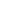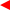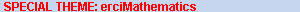contentsERCIM News No.50, July 2002# Spurious Solutions in Finite Element Simulation of Electromagnetic Cavity Resonators

by Paolo Fernandes and Mirco Raffetto

The strange behaviour of some computational models of electromagnetics that produce spurious solutions in addition to physical ones is explained. The necessary and sufficient conditions to avoid these spurious solutions are given.

Wave guides and resonant cavities are basic components of microwave apparatus or particle accelerators. It is thus hardly surprising that the first numerical simulations of these devices were performed a long time ago.

One of the most powerful ways to carry out a numerical simulation of a physical device which can be modelled by a partial differential equation is to approximate that equation by a finite element method. In this kind of method the problem domain is subdivided in cells (elements) and an approximate solution is sought, which is polynomial inside each element and satisfies suitable matching conditions across the common boundaries of adjacent elements. In the case of resonant cavities, this approximate solution is determined by a matrix eigenvalue problem. The eigenvalues give the resonant frequencies while the electromagnetic field is obtained from the components of the eigenvectors (degrees of freedom), whose physical meaning depends on the specific method.

The first finite element models of resonant cavities were developed in the early seventies and it soon became apparent that, together with the resonant modes experimentally observed, many solutions having no counterpart in the real world were also obtained. The resonant frequencies of these ‘spurious modes’ were dramatically dependent on the element size and their field patterns did exhibit very unphysical features (eg, a large divergence, while all physical fields should have been divergence-free). A direct consequence of this difficult situation was that the outputs of numerical simulations were unreliable (as the contamination of a physical mode by a spurious one at the same frequency could not be excluded) or, at least, very difficult to use and inefficient (as, in any case, spurious modes had to be identified and discarded).

A number of attempts to overcome this problem were made, but a satisfactory solution was not proposed until the so-called "edge elements" were found. The degrees of freedom of the most obvious finite elements for vector fields are the value of the field components at specific points on the element boundary and matching these values between adjacent elements makes continuous all field components. Instead, the degrees of freedom of the edge elements are the line integrals of the field along the edges of the elements. Matching them makes continuous across any common face only the components tangential to that face. From the practical viewpoint, these elements made it possible to overcome the drawback of spurious solutions.

Unfortunately, in spite of the enormous number of papers dealing with this spurious mode issue over a period of thirty years, a theory explaining why the edge elements are spurious-free while others are not, was not correctly formulated in this vast literature. In our opinion, this state of affairs had the adverse practical consequence that new finite element models could only be developed without knowing in advance whether they would be spurious-free or not. In our study of these results, we have identified the flaws of the previous theories and we have given counterexamples to them. Quite surprisingly, we found, in particular, that even a satisfactory definition of spurious-free approximation was still lacking.

We have devoted a great deal of research effort to this issue in order to finally obtain a fully satisfactory solution of this basic theoretical problem of computational electromagnetics, including a good definition of spurious-free approximation. Furthermore, by use of results by Descloux, Nassif and Rappaz on the approximation of the spectrum of non-compact operators, we have developed a comprehensive mathematical theory of the phenomenon of spurious modes.

The above mentioned spectral approximation results played a crucial role in the development of our theory because of the following peculiar situation. Even though correct modelling of a resonant cavity leads to an eigenvalue problem for a compact operator, the resulting model includes a divergence-free constraint, which is difficult to implement in a numerical method. Hence, this constraint is usually dropped. Dropping this constraint has a twofold effect on the eigenproblem: (i) extraneous zero frequency eigensolutions are introduced; (ii) the operator is no longer compact. The idea underlying this approach is then to discard any zero (or near zero) frequency solution of the finite element approximation instead of implementing a difficult constraint. Unfortunately, this is not the whole story because conditions ensuring convergence of the spectral approximation of a compact operator are not sufficient to ensure convergence when the operator is non-compact. Therefore, if suitable additional conditions are not satisfied, the extraneous solutions are spread over the whole spectrum, thus giving rise to spurious modes.

Our theory gives some sets of conditions that are necessary and sufficient to avoid spurious modes and explains the behaviour of the spurious modes observed when not all the necessary conditions are satisfied. With the new theory, most of the edge element models previously used on empirical grounds have been rigorously proved to be spurious-free. Both the theory and these proofs hold under very general assumptions that meet almost all the requirements of engineering practice. Simplifying assumptions — so common in mathematical work, but frequently making theoretical results almost useless to the engineer involved in real applications — are deliberately avoided. The required generality is achieved by exploiting the mathematical framework previously developed by one of the authors (P.F.) with G.Gilardi (Department of Mathematics, University of Pavia, Italy) to deal with realistic assumptions in electromagnetics. Finally, we have proved some lemmas that indicate a few modifications that can be applied to a spurious-free finite element without losing its spurious-free character. By exploiting these lemmas it is possible to develop whole families of finite elements that are spurious-free by construction. Further progress in terms of performance optimization can be obtained through the systematical investigation of these spurious-free computational models.